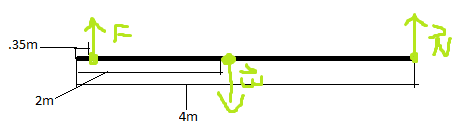# Introductory Torque Statics Problem

## Homework Statement

You're carrying a 4.0-m-long, 24kg pole to a construction site when you decide to stop for a rest. You place one end of the pole on a fence post and hold the other end of the pole 35 cm from its tip.

I've drawn a diagram of the problem on Paint...∑τ=0
τ=F⊥r

## The Attempt at a Solution

I know that ∑τ=F(r1)+w(r2)+N(r3)=0, and I'm going to rewrite it so F(r1)+N(r3)=-w(r2). Now, if I call the left-most point the pivot point, my new equation becomes F(.35m)+4m(9.8 N/kg * 24kg) =-2m(-9.8 N/kg* 24 kg), which simplifies to F(.35m) = -470.4Nm, which makes F=-1344N. This seems way too big to be right; what did I do wrong?